# Solution assignment 10 Fractional functions and graphs

### Assignment 10

Investigate the function: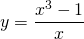and sketch its graph.

### Solution

For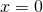we have a vertical asymptote (forthe function becomes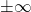). When we try to find a horizontal asymptote we encounter some problems, because for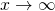orthe graph approaches. In order to get more information of the behavior of the function foror, we rewrite the function as follows: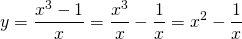We see that the graph approaches: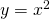whenor. In fact, this parabola is a special asmptote.
It is clear that the graph has no intersection points with the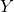-axis (). The intersection point with the-axis can be found by solving the equation: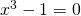We notice immediately that this equation has the solution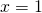and thus we can factorize the function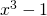: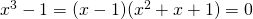The quadratic function has a discriminant which is less than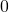is and thus has no solutions. Thus the only intersection point with the-as is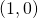.
Because of the special nature of the function we look at it in more detail near. We notice that the second termnearhas either the largest (positive or negative) value: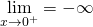andBased on this investigation we can sketch the graph. We also depicted the parabola.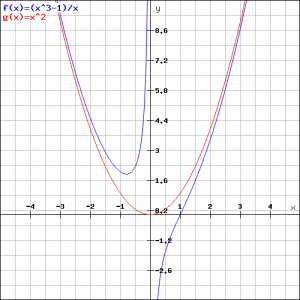0